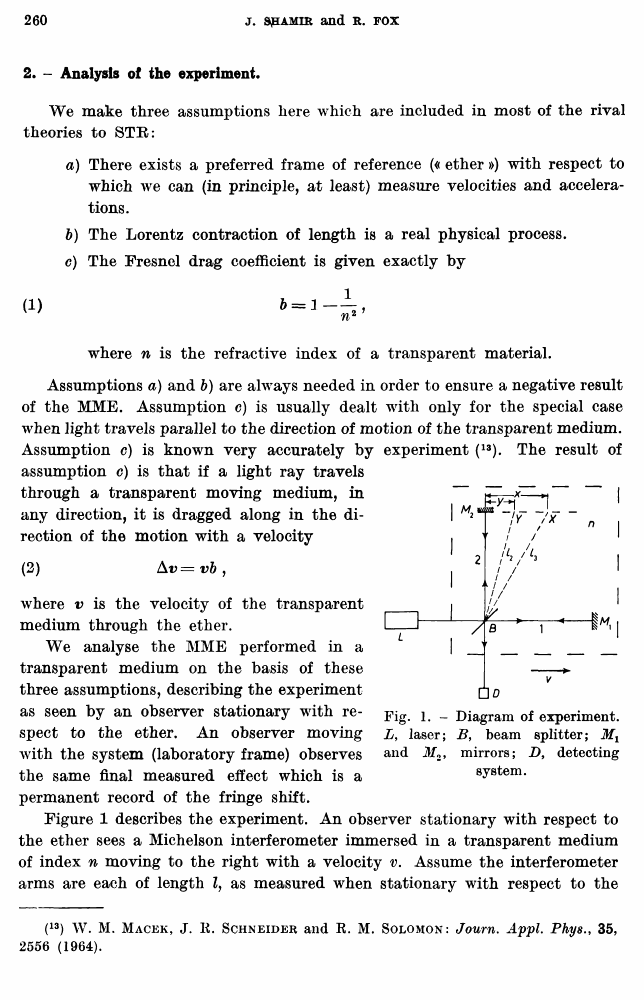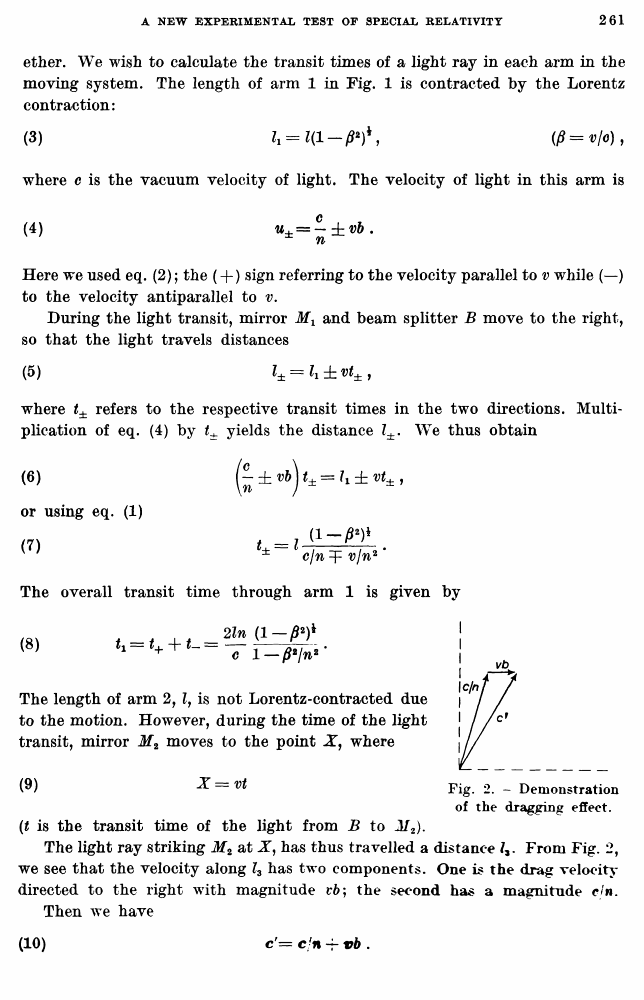Shamir, J.; Fox, R. A new experimental test of special relativity // Nuovo Cimento B Series 10, vol. 62, issue 2, 11 Aug. 1969

В начало   Другие форматы   <<<     Страница 260   >>>258  259  260 261  262  263  264
2. - Analysis of the experiment.

We make three assumptions here which are included in most of the rival theories to STR:

a) There exists a preferred frame of reference (« ether ») with respect to which we can (in principle, at least) measure velocities and accelerations.

b) The Lorentz contraction of length is a real physical process.

c) The Fresnel drag coefficient is given exactly by

where n is the refractive index of a transparent material.

Assumptions a) and b) are always needed in order to ensure a negative result of the MME. Assumption c) is usually dealt with only for the special case when light travels parallel to the direction of motion of the transparent medium. Assumption c) is known very accurately by experiment (13). The result of assumption c) is that if a light ray travels through a transparent moving medium, in any direction, it is dragged along in the direction of the motion with a velocity

(2) Av = vb ,

where v is the velocity of the transparent medium through the ether.

We analyse the MME performed in a transparent medium on the basis of these three assumptions, describing the experiment as seen by an observer stationary with respect to the ether. An observer moving with the system (laboratory frame) observes the same final measured effect which is a permanent record of the fringe shift.

Figure 1 describes the experiment. An observer stationary with respect to the ether sees a Michelson interferometer immersed in a transparent medium of index n moving to the right with a velocity v. Assume the interferometer arms are each of length Z, as measured when stationary with respect to the

(13) W. M. Macek, J. E. Schneider and E. M. Solomon: Journ. Appl. Phys., 35, 2556 (1964).

Fig. 1. - Diagram of experiment. L, laser; B, beam splitter; Mx and Jf2, mirrors; Z>, detecting system.

ether. We wish to calculate the transit times of a light ray in each arm in the moving system. The length of arm 1 in Fig. 1 is contracted by the Lorentz contraction:

where c is the vacuum velocity of light. The velocity of light in this arm is

Here we used eq. (2); the (+ ) sign referring to the velocity parallel to v while (—) to the velocity antiparallel to v.

During the light transit, mirror Mx and beam splitter B move to the right, so that the light travels distances

where t± refers to the respective transit times in the two directions. Multiplication of eq. (4) by t± yields the distance Z±. We thus obtain

(t is the transit time of the light from B to M2).

The light ray striking M2 at X, has thus travelled a distance From Fig. 2, we see that the velocity along l3 has two components. One is the drag velocity directed to the right with magnitude t*6; the second has a magnitude cjn. Then we have

W

(6)

or using eq. (1) (7)

The overall transit time through arm 1 is given by

(8)

The length of arm 2, lf is not Lorentz-contracted due to the motion. However, during the time of the light transit, mirror M2 moves to the point X, where

(9)

X=vt

Fig. 2. - Demonstration of the dragging effect.

(10)

c'= c/n vb .